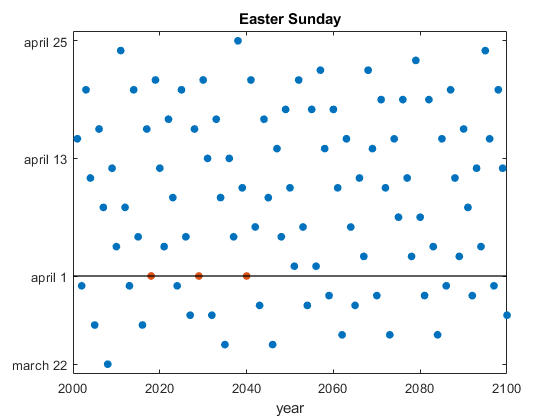# Easter Sunday and April Fools

What do the years 2018, 2029 and 2040 have in common? They are the only years in the 21st century when Easter Sunday occurs on April Fools Day.

### Contents

#### Fool Meets Bunny

    for y = 1:100
april_first = datenum(y+2000,4,1);
delta(y) = easter(y+2000) - april_first;
end
years = 2000 + find(delta == 0)

years =

2018        2029        2040



#### 21st Century

This plot shows the April Fools Easter Sundays in the 21st century and also shows that Easter can occur on any date between March 22 between April 25.

    p = plot(delta,'.');
annotate(p,delta)#### Easter Day

Easter Day is one of the most important events in the Christian calendar It is also one of the most mathematically elusive. In fact, regularization of the observance of Easter was one of the primary motivations for calendar reform. The informal rule is that Easter Day is the first Sunday after the first full moon after the vernal equinox. But the ecclesiastical full moon and equinox involved in this rule are not always the same as the corresponding astronomical events, which, after all, depend upon the location of the observer on the earth. Some details are in my blog post from 2013.

Computing the date of Easter is featured in Don Knuth’s classic The Art of Computer Programming and has consequently become a frequent exercise in programming courses.

Wikipedia has several relevant articles. Easter, Computus, and Metonic_cycle.

#### Easter function

Here is the Easter function from Experiments in MATLAB. It is also available here.

    % addpath ../exm
type easter

function dn = easter(y)
% EASTER  Date of Easter.
% EASTER(y) is the datenum of Easter in year y.
% Ref: Donald Knuth, The Art of Computer Programming,
%      Fundamental Algorithms, pp. 155-156.

%   Copyright 2014 Cleve Moler
%   Copyright 2014 The MathWorks, Inc.
% Golden number in 19-year Metonic cycle.
g = mod(y,19) + 1;

% Century number.
c = floor(y/100) + 1;

% Corrections for leap years and moon's orbit.
x = floor(3*c/4) - 12;
z = floor((8*c+5)/25) - 5;

% Epact.
e = mod(11*g+20+z-x,30);
if (e==25 && g>11 || e==24), e = e + 1; end

% Full moon.
n = 44 - e;
if n < 21, n = n + 30; end

% Find a Sunday.
d = floor(5*y/4) - x - 10;

% Easter is a Sunday in March or April.
d = n + 7 - mod(d+n,7);
dn = datenum(y,3,d);


Published with MATLAB® R2020a

|

### コメント

コメントを残すには、ここ をクリックして MathWorks アカウントにサインインするか新しい MathWorks アカウントを作成します。